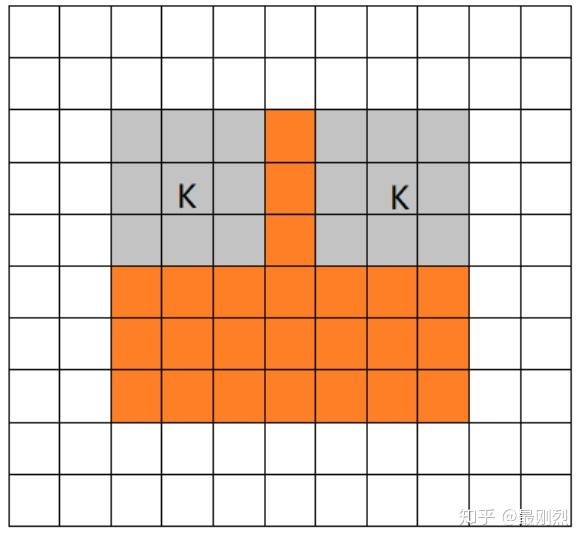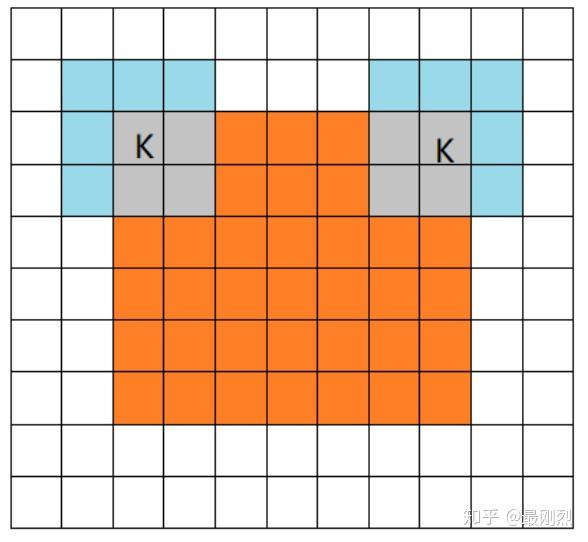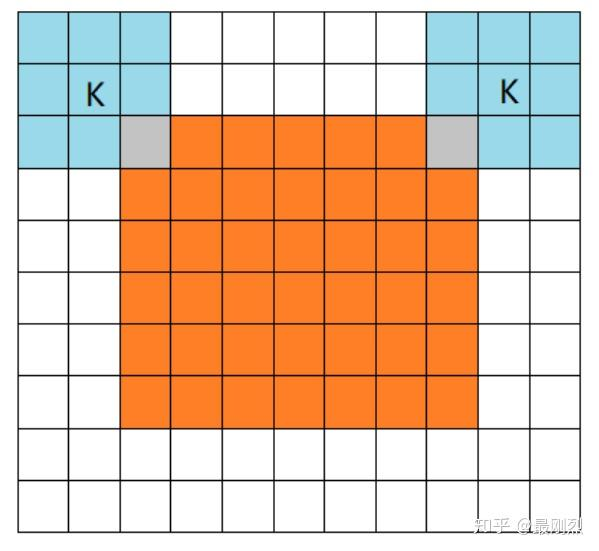## Valid Mode• 输入图片大小: W*W

• Filter大小:

• 步长: S

N = (W- F+ 2P)/S+1

$$n_{\text {output}}=\left[\frac{n_{\text {input}}-f+1}{s}\right]$$

conv2d的VALID方式不会在原有输入的基础上添加新的像素（假定我们的输入是图片数据，因为只有图片才有像素），输出矩阵的大小直接按照公式计算即可。

## Same Mode$$n_{\text {output}}=\left[\frac{n_{\text {input}}}{s}\right]$$

pad_needed_height = (new_height [Dash] 1) * S + F - W